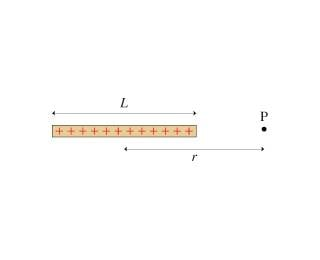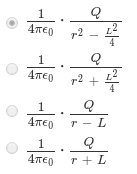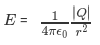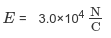# Find an expression for the electric field(Mastering Physics)

I just wanted to post a solution for anyone interested. Any notes on how to solve would be nice for others.

1. Homework Statement

Mastering Physics (3rd edition) Problem 26.40

The figure (Figure 1) shows a thin rod of length L with total charge Q.Part A) Find an expression for the electric field strength on the axis of the rod at distance r from the center.

Part B) Verify that your expression has the expected behavior if r≫L.
Express your answer in terms of variables Q, rand constants π, ε0.

Part C) Evaluate E at r = 3.6cm if L = 5.0 cm and 2.2nC .
Express your answer to two significant figures and include the appropriate units.

3. The solution
Part A)Part B)Part C)